# Discrete-time Fourier transform (DTFT)

In the last two posts in my Fourier transform series I discussed the continuous-time Fourier transform. Today I want to start getting "discrete" by introducing the discrete-time Fourier transform (DTFT).

The DTFT is defined by this pair of transform equations: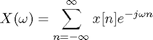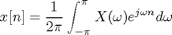Here x[n] is a discrete sequence defined for all n: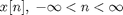I am following the notational convention (see Oppenheim and Schafer, Discrete-Time Signal Processing) of using brackets to distinguish between a discrete sequence and a continuous-time function. n is unitless. The frequency-domain variable,, is continuous with units of radians.

Note that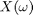is periodic with period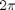.

Here are a few common transform pairs:

Unit Impulse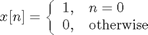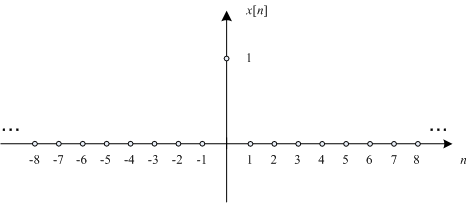DTFT of Unit Impulse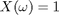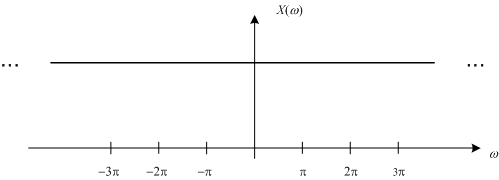Rectangular Pulse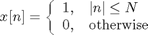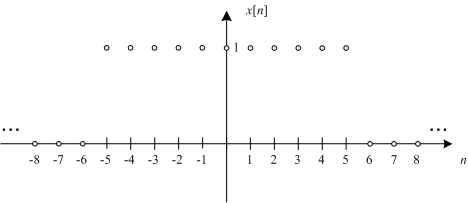DTFT of Rectangular Pulse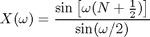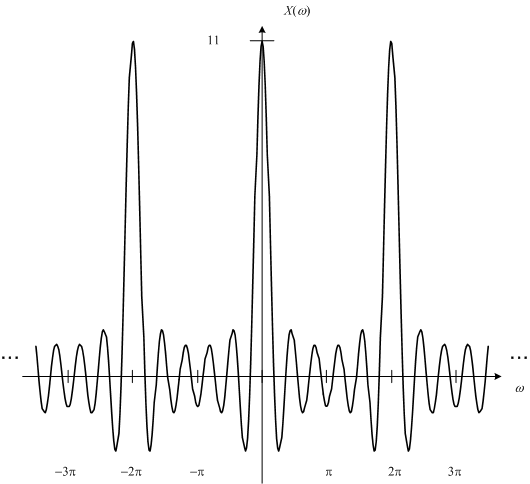Note that the DTFT of a rectangular pulse is similar to but not exactly a sinc function. It resembles the sinc function betweenand, but recall thatis periodic, unlike the sinc function.

Cosine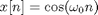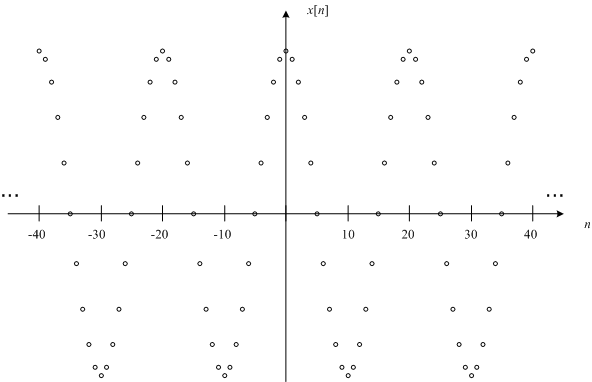DTFT of Cosine

The DTFT of a discrete cosine function is a periodic train of impulses: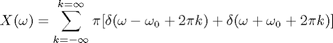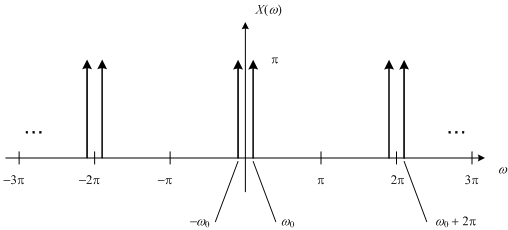I updated the above plot on 6-Jan-2010 to show the location of the impulses. -SE

Because of the periodicity ofit is very common when plotting the DTFT to plot it over the range of just one period: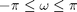. For example, the DTFT of the rectangular pulse will most often be shown like this: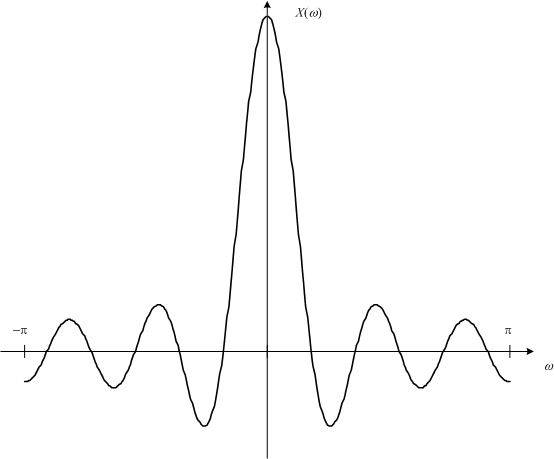Next time I'll discuss the relationship between the continuous-time and the discrete-time Fourier transforms. Until then, Happy New Year everyone!

Published with MATLAB® 7.9

|

### コメント

コメントを残すには、ここ をクリックして MathWorks アカウントにサインインするか新しい MathWorks アカウントを作成します。